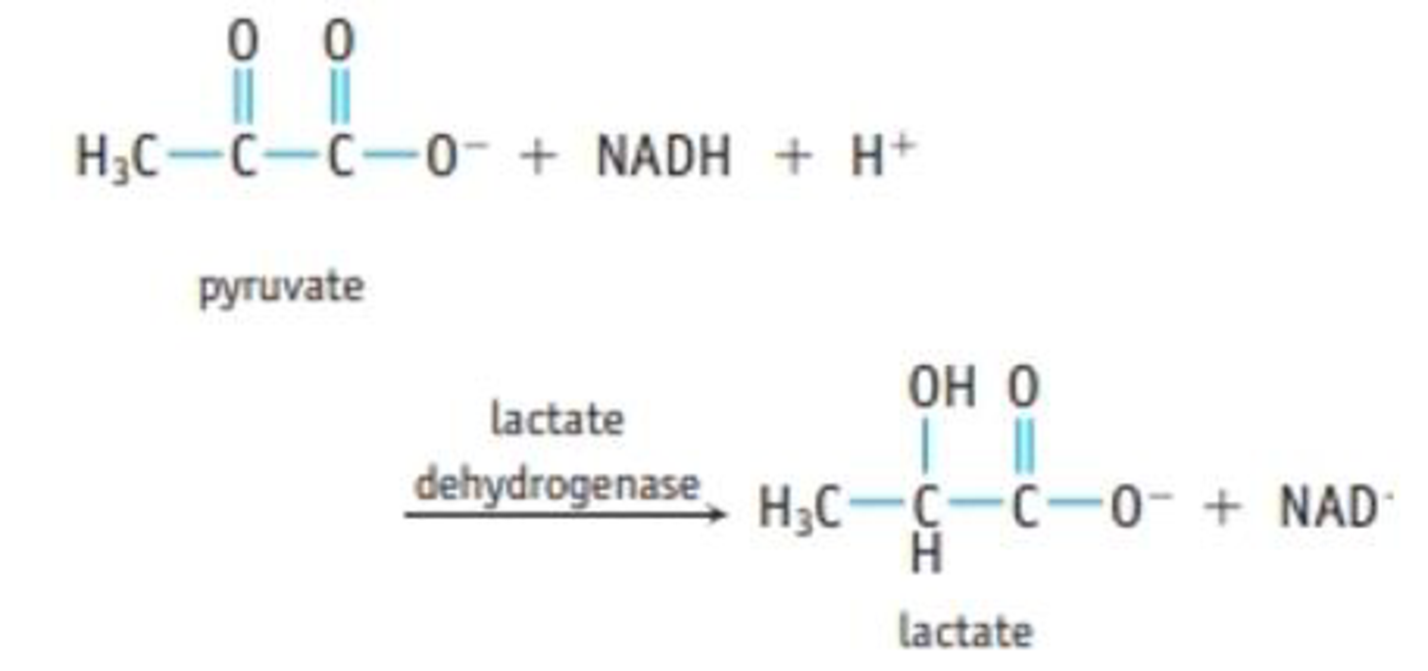# Muscle cells need energy to contract. One biochemical pathway for energy transfer is the breakdown of glucose to pyruvate in a process called glycolysis . In the presence of sufficient oxygen in the cell, pyruvate is oxidized to CO 2 and H 2 O to make further energy available. However, under extreme conditions not enough oxygen can be supplied to the cells, so muscle cells produce lactate ion according to the reaction where Δ r G °′ = −25.1 kJ/mol. In living cells, the pH value is about 7. The hydronium ion concentration is constant and is included in Δ G °, which is then called Δ r G °′ ( Applying Chemical Principles 18.1 Thermodynamics and Living Things ). ( This problem is taken from the problems for the 36th International Chemistry Olympiad for high school students held in Kiel Germany in 2004 .) (a) Calculate Δ r G ° for the reaction at 25 °C. (b) Calculate the equilibrium constant K ′ . (The hydronium ion concentration is included in the constant. That is, K′ = K · [H 2 O + | for the reaction at 25 °C and pH = 7.0.) (c) Δ r G ° is the free energy change under standard conditions; that is, the concentrations of all reactants (except H 3 O + are 1.00 mol/L. Calculate Δ r G ° at 25 °C, assuming the following concentrations in the cell: pyruvate, 380 μ mol/L; NADH, 50 μ mol/L; lactate ion, 3700 μ mol/L; and NAD + ion, 540 μ mol/L.### Chemistry & Chemical Reactivity

9th Edition
John C. Kotz + 3 others
Publisher: Cengage Learning
ISBN: 9781133949640

#### Solutions

Chapter
Section### Chemistry & Chemical Reactivity

9th Edition
John C. Kotz + 3 others
Publisher: Cengage Learning
ISBN: 9781133949640
Chapter 18, Problem 88SCQ
Textbook Problem
253 views

## Muscle cells need energy to contract. One biochemical pathway for energy transfer is the breakdown of glucose to pyruvate in a process called glycolysis. In the presence of sufficient oxygen in the cell, pyruvate is oxidized to CO2 and H2O to make further energy available. However, under extreme conditions not enough oxygen can be supplied to the cells, so muscle cells produce lactate ion according to the reactionwhere ΔrG°′ = −25.1 kJ/mol. In living cells, the pH value is about 7. The hydronium ion concentration is constant and is included in ΔG°, which is then called ΔrG°′ (Applying Chemical Principles 18.1 Thermodynamics and Living Things). (This problem is taken from the problems for the 36th International Chemistry Olympiad for high school students held in Kiel Germany in 2004.) (a) Calculate ΔrG° for the reaction at 25 °C. (b) Calculate the equilibrium constant K′. (The hydronium ion concentration is included in the constant. That is, K′ = K · [H2O+| for the reaction at 25 °C and pH = 7.0.) (c) ΔrG° is the free energy change under standard conditions; that is, the concentrations of all reactants (except H3O+ are 1.00 mol/L. Calculate ΔrG° at 25 °C, assuming the following concentrations in the cell: pyruvate, 380 μmol/L; NADH, 50 μmol/L; lactate ion, 3700 μmol/L; and NAD+ ion, 540 μmol/L.

(a)

Interpretation Introduction

Interpretation:

The ΔrGo for given reaction should be calculated.

Concept introduction:

The cells in our body requires energy to do its function. This energy is provided by the breakdown of glucose to pyruvate. The process is known as glycolysis. Pyruvate generated gives CO2 and H2O in presence of oxygen. However, if the oxygen supply is limited then lactate formation takes place. The reaction is written as,

### Explanation of Solution

The ΔrGo for the process of conversion of pyruvate to lactate is calculated below.

Given:

The value of ΔrGo is 25.1 kJ/mol.

The pH of the living cell is 7 and it is related to hydrogen ion concentration by the formula,

pH=log[H+]7=log[H+]

Thus,

[H+]=1×107

ΔrGo is related to the ΔrGo by the expression,

ΔrGo'rGo+2

(b)

Interpretation Introduction

Interpretation:

The K' for the given reaction should be calculated.

Concept introduction:

The cells in our body requires energy to do its function. This energy is provided by the breakdown of glucose to pyruvate. The process is known as glycolysis. Pyruvate generated gives CO2 and H2O in presence of oxygen. However, if the oxygen supply is limited then lactate formation takes place. The reaction is written as,

(c)

Interpretation Introduction

Interpretation:

The ΔrG' for the given reaction under given temperature conditions should be calculated.

Concept introduction:

The cells in our body requires energy to do its function. This energy is provided by the breakdown of glucose to pyruvate. The process is known as glycolysis. Pyruvate generated gives CO2 and H2O in presence of oxygen. However, if the oxygen supply is limited then lactate formation takes place. The reaction is written as,

### Still sussing out bartleby?

Check out a sample textbook solution.

See a sample solution

#### The Solution to Your Study Problems

Bartleby provides explanations to thousands of textbook problems written by our experts, many with advanced degrees!

Get Started

Find more solutions based on key concepts
Recommendations about carbohydrate intake can seem to be contradictory. On one hand, it is recommended that the...

Nutrition: Concepts and Controversies - Standalone book (MindTap Course List)

Why is there a mass-luminosity relation?

Horizons: Exploring the Universe (MindTap Course List)

What are sister chromatids?

Human Heredity: Principles and Issues (MindTap Course List)

A car of mass m moving at a speed v1 collides and couples with the back of a truck of mass 2m moving initially ...

Physics for Scientists and Engineers, Technology Update (No access codes included)

Classify the amines from Question 76 as primary, secondary, or tertiary.

Introductory Chemistry: An Active Learning Approach

What is a meteorological tide?

Oceanography: An Invitation To Marine Science, Loose-leaf Versin